## Example Questions

← Previous 1 3 4

### Example Question #1 : Even / Odd Numbers

The sum of seven consecutive even integers is 0.

Column A: The product of the seven integers

Column B: 2

Column B is greater.

The relationship cannot be determined from the given information.

The two quantities are equal.

Column A is greater.

Column B is greater.

Explanation:

For the sum of 7 consecutive even integers to be zero, the only sequence possible is –6, –4, –2, 0, 2, 4, 6. This can be determined algebraically by assigning the lowest number in the sequence to be “y” and adding 2 for each consecutive even integer, and then setting this equal to zero.

y, y + 2, y + 4, y + 6 . . .

The product of any number and 0 is 0.

Therefore, column B must be greater.

### Example Question #2 : Even / Odd Numbersand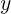are both even whole numbers.

What is a possible solution for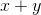?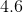Explanation:

Ifandare both even whole numbers, then their addition must be an even whole number as well. Althoughis an even number, it is not a whole number and could therefore not be a solution. This means the only possible solution would be.

### Example Question #3 : Even / Odd Numbers

If John buys twenty-two apples on Monday, and thirty-four bananas on Tuesday, how many pieces of fruit does John have?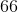Explanation: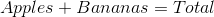Plugging in the values given we arrive at the total fruit John has: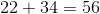A good note about adding even numbers--any even numbers--is that if you add two even numbers, their sum will ALWAYS be an even number.

### Example Question #4 : Even / Odd Numbers

Choose the answer below which best solves the following problem: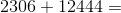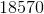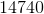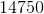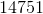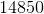Explanation: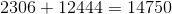To deal with a problem with this many digits, often the best strategy is to line up one number over the other, then add the places one at a time.  Don't forget to carry a one every time the addition goes over ten.  Also, note that any time you add two even numbers, their sum will ALWAYS be an even number.

### Example Question #5 : Even / Odd Numbers

Assumeandare both even whole numbers and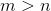.

What is a possible solution for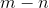?Explanation:

Sincemust result in a positive whole number. The only answer that fits these requirements of being both positive and whole number is.

### Example Question #6 : Even / Odd Numbers

A bus has sixteen passengers at its first stop.  It drops off three at the second stop, and three at the third stop.  At the fourth stop, eveyone else gets off the bus.  How many people got off at the fourth stop?Explanation:

First, add the total number of passengers that got off BEFORE the fourth stop.  Three plus three is six, so you know that you've lost six total passengers before the fourth stop.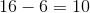, so there are ten passengers remaining at the fourth stop, and that's how many get off there.

### Example Question #7 : Even / Odd Numbers

Choose the answer below which best solves the following equation: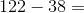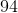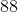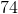Explanation:

If it's simpler for you, you can split this problem into two parts:  First, takeaway from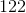, and you're left with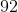.  Then, take awayfrom(you can even count backwards if necessary), and you'll be left with the final answer,.

### Example Question #8 : Even / Odd Numbers

If m, n and p are odd integers, which of the following must be an odd integer?

m + n + p + 1

m * p * (n -1)

m * (n + p)

(m - 2 )* n * p

(m + 1) * n

(m - 2 )* n * p

Explanation:

When multiplying odd/even numbers, we know that odd * odd = odd, and odd * even = even. We also know that odd + odd = even. We will proceed to evaluate each answer choice, knowing that m, n and p are odd.

(m + 1) * n

m + 1 becomes even. This gives us even * odd = even.

m + n + p + 1

Odd + odd + odd + odd = even + odd + odd = even + even = even.

(m - 2 )* n * p

m - 2 is stil odd. This gives us odd * odd * odd = odd * odd = odd.

m * (n + p)

Odd + odd is even, so here we have odd * even = even.

m * p * (n -1)

n - 1 becomes even so we have odd * odd * even = odd * even = even.

The correct answer is therefore m * p * (n -1).

### Example Question #9 : Even / Odd Numbers

The operation ¤ is defined for all integers x and y as: x ¤ y = 4x-y2.

If x and y are positive integers, which of the following cannot produce an odd value?

x ¤ (y+1)

x ¤ y2

y ¤ x

x ¤ y

x ¤ 2y

x ¤ 2y

Explanation:

For this problem, we must recognize under what arithmetic conditions an even or odd number is produced. We do not know what the values for x and y are, but we do know for example that any two numbers added can be either even or odd, and and any number multiplied by two must be even (e.g. 2 * 2 = 4; 3 * 2 = 6), or an odd number multiplied by another odd is always even.

In this situation, we have to ensure that an arithmetic operation (subtraction) must result only in an even number, by further ensuring that the variables themselves are fixed to either odd or even values.

In this situation, we know that due to the "*2 (= 2 * 2)" principle, the 4x component always being an even value. The only situation in which the operation "4x – y2" will be even is if the "y2" term is also even, since an even minus an odd would be odd. The only situation in which the "y2" term is even is if the y itself is even, since an odd number squared always results in an odd value (e.g. 3= 9; 7= 25). To ensure that the y itself is even, we must also double it to "2y"(2).

We cannot add 1 to a random variable y as it may still result in an odd y-value. Similarly, since we already stated that a squared value may still be odd, we cannot be sure that squaring the y will also result in an even number.

### Example Question #1 : Even / Odd Numbers

What is the 65th odd number?

131

127

133

129

125

129

Explanation:

Doing this by counting the odd numbers will take way too long for the GRE.  And if you look at the answer choices, you see five consecutive odd numbers, so one little mistake in counting will give you the wrong answer!  Instead, we should use the formula for finding odd numbers: the nth odd number is 2n – 1.  (The nth even number is 2n.)  So the 65th odd number is 2 * 65 – 1 = 129.

← Previous 1 3 4

Tired of practice problems?

Try live online GRE prep today.